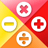# AlgebraQuantitative Aptitude Questions and Answers section on “Algebra” with solution and explanation for competitive examinations such as CAT, MBA, SSC, Bank PO, Bank Clerical and other examinations.

# Aptitude Question ID : 93836

If , then x equals : [A] [B] [C] [D] Show Answer Given Expression, or x = 0 given indeterminate value. Hence option [B] is the right answer.

# Aptitude Question ID : 93832

If a = 7, b = 5 and c = 3, then the value of is: [A]0 [B]8 [C]-12 [D]12 Show Answer 12 Given Expression, Hence option [D] is the right answer.

# Aptitude Question ID : 93829

If x = 0.5 and y = 0.2, then value of is equal to : [A]1.3 [B]1.1 [C]0.5 [D]0.6 Show Answer 0.6 Given that, x = 0.5 and y = 0.2 Now we have to find the value of putting on values of x and y Hence option [D] is the right answer.

# Aptitude Question ID : 93824

Find out the value of [A] [B] [C] [D] Show Answer 9 Given Expression, Hence option [C] is the right answer.

# Aptitude Question ID : 93820

If , then what would be the value of x : [A]36 [B]37 [C]32 [D]31 Show Answer 36 The given expression is, to 30 terms Hence option [A] is the right answer.